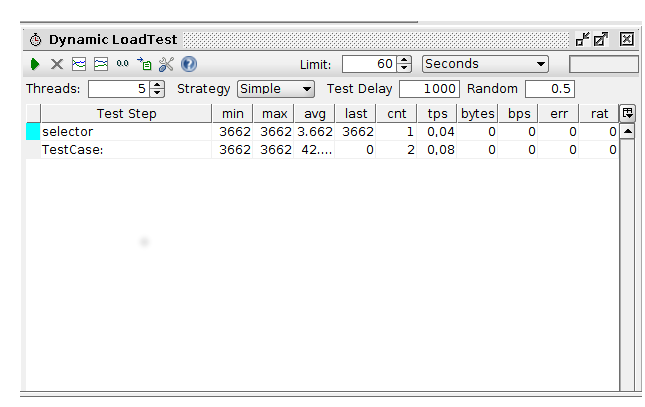Kategorien

# DEV-NOTES|SoapUI: Run random tests in a load test

Sometimes it is useful to set up a SoapUI test for simulating different usage scenarios randomly. The following solution provides a random selection from prepared test steps resulting in a different behavior of the tested service.

Setting up the test environments includes three steps:

1. Create test suite containing test cases for each desired scenario2. Create test suite with one test case which contains at least one groovy test step for the dynamic test step selection containing the following code:
```import org.apache.log4j.Level
import java.util.Random

// just for debuging - change to Level.SEVERE for actually running the test
log.setLevel(Level.DEBUG)
log.debug("selecting a test case")

// get project for test case for later test step selection
def project = testRunner.getTestCase().getTestSuite().getProject()
log.debug("Project: "+project)

// select test suite which provides the prepared test step
def suite = project.getTestSuiteByName("CalculatorService TestSuite")

// -- only for debugging: print test cases
def allTestCases = suite.getTestCaseList()
for(def tc : allTestCases) {
log.debug("\t Test Case: "+ tc.getName())
}

// select test case from which the test steps are taken
def selectedTestCases = allTestCases

// randomly select test step by index from the given test suite
// you might adjust the behavior by modifying the probability of
// the resulting index
def idx = Math.abs( (new Random()).nextInt() % selectedTestCases.size())
log.debug("selected index: "+idx)

// select test based on randomly calculated index
def selectedTest = selectedTestCases.get(idx)
log.debug("Running test: "+ selectedTest.getName())

// execute test
def result = selectedTest.run(context.getProperties(), false)
//log.debug("Test result is: "+ result.getStatus())

// propagate result back to the originating test step
return result

```
3. Set up load test using the „dynamic“ test case for simulating different users behavior.The basic idea is selecting the test step based on the randomly calculated index from a list of test cases provided by a dedicated test suite. The given example facilitates a simple model for index selection, but it is expandable to model more sophisticate algorithms for index calculation. One example for a slightly improved might by the calculation of a weighted index. This might be useful if the expected characteristics are known, like testing an query service supposed to have about 60% of all requests being no hits.

Running the load test based on the dynamic selection offers a facile opportunity of modeling a more user like behavior testing the service implementation tailored to the expected characteristics for given scenarios.